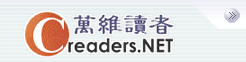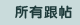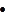设万维读者为首页广告服务技术服务联系我们关于万维简体繁体手机版
 首页 新闻 论坛 博客 视频 分类广告 购物版主：
 五 味 斋 茗香茶语 天下论坛 竞技沙龙 彩虹之约 摄友部落 诗词歌赋 七荤八素 高山流水 海 二 代 教育学术 笑林之声 健康生活 史地人物 军事天地 跨国婚姻 恋恋风尘 灵机一动 股市财经 加国移民 流行前线 新 大 陆 影视娱乐 焦点房谈 我爱我车 美国移民
 万维读者网 > 灵机一动 > 跟帖
 p(1)=4 送交者: zhf 2019月11月02日22:06:16 于 [灵机一动] 发送悄悄话 回  答: 趣味的数学-142 由 gugeren 于 2019-11-02 15:46:28 p(x) = x^2 + bx + cf(x)=x^4 + 6x^2 + 25 g(x)=3x^4 + 4x^2 + 28x + 5f(x)=x^4 + 10x^2 + 25 - 4x^2 = (x^2+5)^2 - 4x^2 = (x^2 - 2x + 5)(x^2 + 2x + 5)p(x)一定是f(x)的两个因子之一。f(1)=(4)(8)g(1)=(4)(10)所以p(1)=4
 0%(0)
 0%(0)是　/无内容 - gugeren 11/03/19 (0)实用资讯
 一周点击热帖 更多>>
 一周回复热帖
 历史上的今天：回复热帖
 2017: 物理附加题五：如下图所示，有两个完全 2017: 《将军在上》马思纯与王楚然配一脸 2014: 不转动的陀螺挂在导读好长时间了，我试